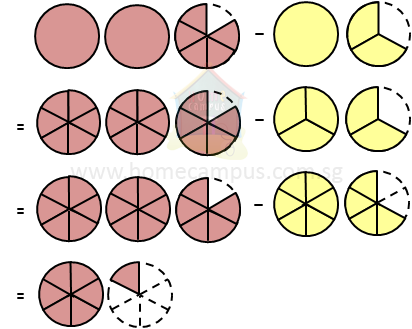## How to Subtract Mixed Numbers and Fractions?

Subtract Fractions Calculator

#### 1. The video below explains how to subtract mixed numbers.

A mixed number or mixed fraction has two parts: a whole number and a proper fraction.

Mixed number  =  whole number  +  proper fraction

#### 2. I have 2 5 6 pepperoni pizzas and 1 2 3 cheese pizzas. How many more pepperoni pizzas do I have than cheese pizzas?

Pepperoni pizzas  =>
 2 5 6

Cheese pizzas  =>
 1 2 3

Pepperoni  −  Cheese  =>
 2 5 6
−
 1 2 3
=  ?2 5 6
−
 1 2 3

 (2 x 6) + 5 6
−
 (1 x 3) + 2 3
(First, change both mixed numbers to improper fractions)
 17 6
−
 5 3

 17 x 1 6 x 1
−
 5 x 2 3 x 2
(Second, change the fractions to like fractions)
 17 6
−
 10 6
(Next, subtract the fractions)
 7 6

=  1 +
 1 6
(Finally, change the improper fraction to mixed number)
 1 1 6

I have
 1 1 6
more pepperoni pizzas than cheese pizzas.

#### 3. What is 3 1 2  −  1 5 ?

 3 1 2
−
 1 5

 (3 x 2) + 1 2
−
 1 5
(change mixed number to improper fraction)
 7 2
−
 1 5

 7 x 5 2 x 5
−
 1 x 2 5 x 2
(change to like fractions)
 35 10
−
 2 10

 35 - 2 10

 33 10

=  3 +
 3 10
(change the improper fraction to mixed number)
 3 3 10